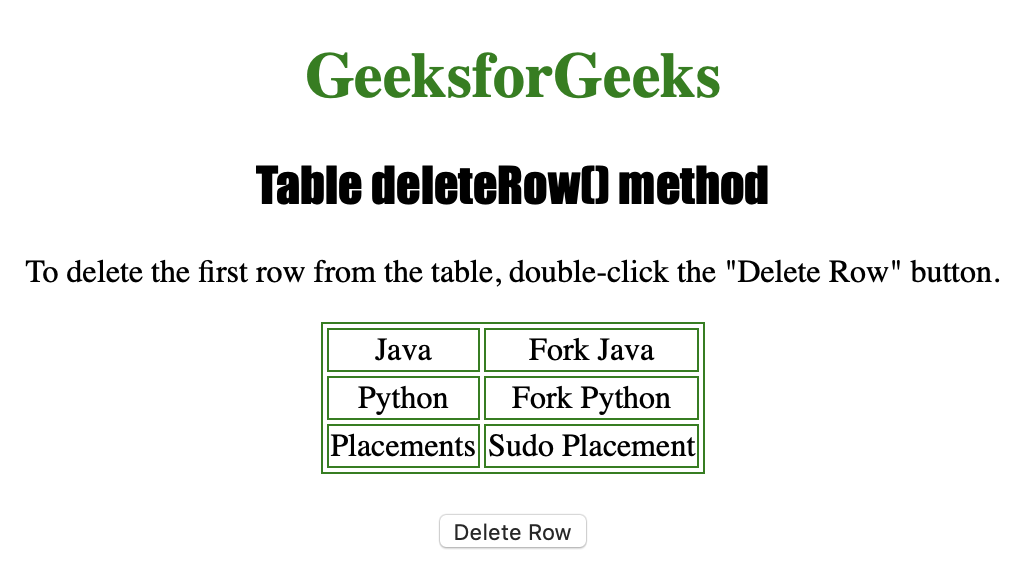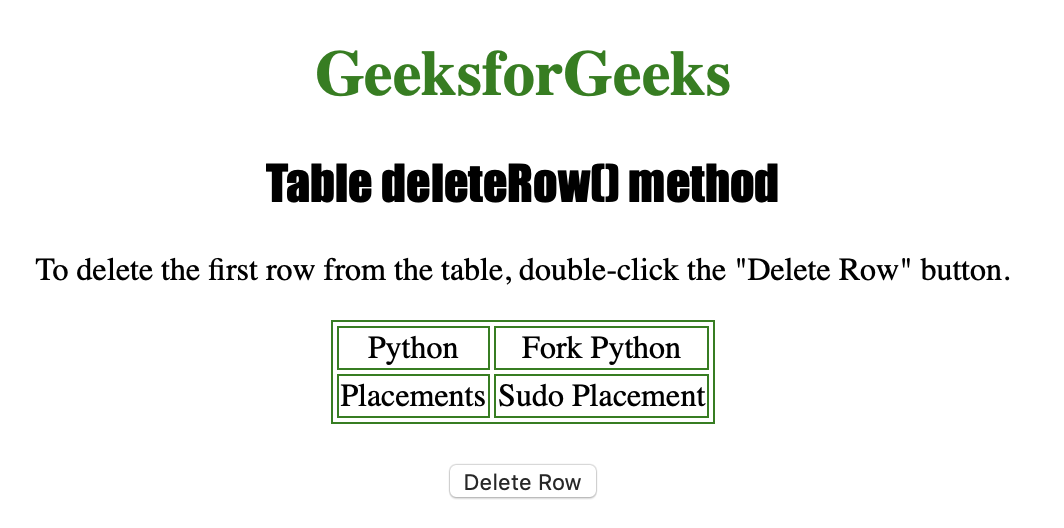Related Articles
HTML | DOM Table deleteRow( ) Method
• Last Updated : 19 Feb, 2019

The Table deleteRow() method is used for removing a <tr> element from a table. In other words, Table deleteRow() method is used for deleting row(s) at the specified index in the table.

Syntax

`tableObject.deleteRow(index)`

Parameters Used

• index :It is used to specify the position of the row to be deleted. The value 0 results in the deletion of the first row whereas -1 can be used to delete the last row.

Below program illustrates the Table deleteRow() method :
Example-1: Deleting the first row from a table.

 ` ` `<``html``> ` ` `  `<``head``> ` `    ``<``title``>Table deleteRow() method in HTML ` `    ``<``style``> ` `        ``table, ` `        ``td { ` `            ``border: 1px solid green; ` `        ``} ` `         `  `        ``h1 { ` `            ``color: green; ` `        ``} ` `         `  `        ``h2 { ` `            ``font-family: Impact; ` `        ``} ` `         `  `        ``body { ` `            ``text-align: center; ` `        ``} ` `    `` ` ` ` ` `  `<``body``> ` ` `  `    ``<``h1``>GeeksforGeeks ` `    ``<``h2``>Table deleteRow() method ` ` `  `    ``<``p``>To delete the first row from the table, ` `      ``double-click the "Delete Row" button. ` ` `  `    ``<``table` `id``=``"Courses"` `align``=``"center"``> ` `        ``<``tr``> ` `            ``<``td``>Java ` `            ``<``td``>Fork Java ` `        `` ` `        ``<``tr``> ` `            ``<``td``>Python ` `            ``<``td``>Fork Python ` `        `` ` `        ``<``tr``> ` `            ``<``td``>Placements ` `            ``<``td``>Sudo Placement ` `        `` ` ` `  `    `` ` `    ``<``br``> ` ` `  `    ``<``button` `ondblclick``=``"row()"``> ` `      ``Delete Row ` `  `` ` ` `  `    ``<``script``> ` `        ``function row() { ` `           `  `          ``// delete row (index-0). ` `          ``document.getElementById("Courses").deleteRow(0); ` `        ``} ` `    `` ` ` `  ` ` ` `  ` `

Output:After clicking the buttonSupported Browsers:

• Apple Safari
• Internet Explorer
• Firefox